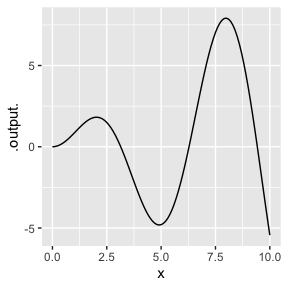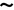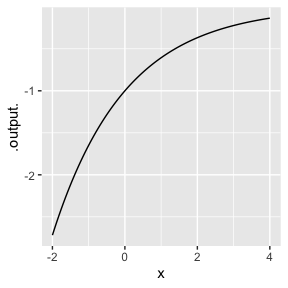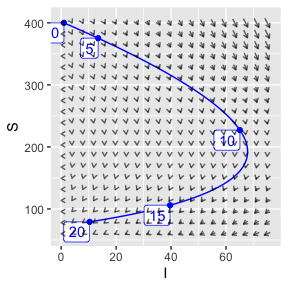# Beginners’ Guide to Calculus with R

#### August 2022

This guide is for calculus students or instructors who are beginners with R. It describes an “R package” called {mosaicCalc} which provides software with a unified and consistent user interface for calculus operations. {mosaicCalc} is the engine behind the MOSAIC Calculus textbook, available free online. However, instructors can and do use {mosaicCalc} independently of MOSAIC Calculus.

Many R packages provide narrative documentation in the form of “vignettes,” the word used in the R community for guides such as this one. This guide is one of three vignettes for {mosaicCalc}. There is also a quick-reference vignette which may be helpful for those who need to jog their memory when writing {mosaicCalc} commands. Finally, a third vignette explains some features and syntax of {mosaicCalc} that an experienced R user may not have previously encountered.

As you may know, R is an environment and language for technical computing. Think of technical computing as serving the specialized needs of scientists and engineers. R is most widely associated with data science and statistics, but it is also well suited to calculus and many other diverse fields. This results, in part, from the “open source” nature of the R environment. An aspect of “open source” is that anyone can contribute new capabilities to the environment using an officially standardized format called an “R package.” There are thousands of such packages available through the official distribution network called CRAN. {mosaicCalc} is one of those packages and works in conjunction with other CRAN packages.

As you can see from the previous two paragraphs, we use a monospaced font when referring to names that are part of the computer system. Many of the names you will encounter, like D(), antiD(), and Integrate(), will be names of “functions” that allow you to perform computations such as differentiation and integration. {mosaicCalc} is not a function. Rather it is a collection of functions that inter-operate and follow similar conventions. The curly braces in {mosaicCalc} remind readers that it is a collection of functions.

{mosaicCalc}, like all R packages, needs to be installed before it can be used. Often, instructors arrange to have packages installed automatically. You can check by giving the R command library(mosaicCalc). If the command works, the installation has already been done. Otherwise, the command will produce an error message (check your spelling carefully!) if you need to install {mosaicCalc} and some related packages. In this case, check with your instructor if there is a specific process for your educational institution. (An example: “Use rstudio.cloud.”) Otherwise, you can carry out the installation using the setup instructions in the MOSAIC Calculus textbook via this link.

Once you have started a session in the R system, you need to direct R to provide the {mosaicCalc} software. Do this with the following directive:

library(mosaicCalc)

## The {mosaicCalc} command pattern

A “command” is a complete instruction to the computer to perform a specified task. There are only about a dozen commonplace tasks used in calculus. To begin, consider the familiar task of drawing a graph of a mathematical function. A function-graphing command needs to include at least three pieces of information.

1. Which type of graph to make.
2. What mathematical function to graph.
3. The bounds for the input(s) to the function.

Constructing a command means laying out this information in a manner the computer can make sense of. Most {mosaicCalc} commands follow a consistent pattern. For instance,

slice_plot(x*sin(x) ~ x, bounds(x = 0:10))The underlying pattern becomes more evident if we divide the command into parts, one for each piece of information.

$\underbrace{\mathtt{slice\_plot}}_\text{task name}{\color{magenta}{\left(\strut\right.}}\ \underbrace{\mathtt{x*sin(x)\ {\verb+~+}\ x}}_\text{function description}\ \color{magenta}{\LARGE,}\ \ \underbrace{\mathtt{bounds(x = 0\!:\!5)}}_\text{bounds on input to function}\color{magenta}{\left.\strut\right)}$

The command includes punctuation which, like punctuation in English, marks boundaries between elements. There are three elements in this command:

The task name is the first element of every {mosaicCalc} command, and it is always followed by an opening parenthesis. The corresponding closing parenthesis goes at the very end of the command.

Almost always, the function description is the second element of a {mosaicCalc} command, following the opening parenthesis. The function description is written in an R format called a “tilde expression,” which is described in the next section of this document.

Graphics commands always have a third element—the “bounds”— specifying the region over which to draw the graph. Note that a comma separates the second and third elements of the command.

Commands occasionally include additional elements to give more details, such as the color of the graph. These are always separated from the previous elements by a punctuating comma.

You will encounter new calculus tasks as you progress through a calculus course. Each such task has a specific {mosaicCalc} task name. But the commands you construct to perform these tasks will always have a format similar to the example above. For instance, there is a calculus task called “anti-differentiation,” which can be carried out with a command starting with antiD(), for instance:

antiD(x*sin(x) ~ x)
## function (x, C = 0)
## sin(x) - x * cos(x) + C

Even if you don’t know what “anti-differentiation” is, you should be able to make sense of the structure of the command: a task name followed by a function description with the appropriate punctuation. The computer produces an output in response to the command. In the slice_plot() example, the output is a graph. In the antiD() example, the output is a new mathematical function.

## Tilde expressions

An expression in computing is more or less like a sentence, phrase, or word in English: a meaningful unit of communication. A tilde expression is a type of statement in the R language that involves the character called “tilde,” written as. Here are some examples of tilde expressions, which are used in {mosaicCalc} mainly to specify mathematical functions. Some examples:

• a*x^2 + b*x + c ~ x
• f(2*t) ~ g(t)
• dz ~ c*z - b*x*y
• exp(-k*t) ~ .

Note that each of these expressions is centered on the tilde character. (Sometimes, you have to look very closely to distinguish tilde ~ from the minus sign -.) There is always a right-hand side, which might be long or very short as in the . in the tilde expression exp(-k*t) ~ .

Many newcomers misinterpretas meaning “equals.” But in realitysimply marks an expression as special. Special in what way? You’ll come to understand that as you gain experience with tilde expressions. As a preview, which you may not understand at this point, consider exp(-k*t) ~ . which has a period to the right of the tilde. Without the ~ . that expression would be simply exp(-k*t) which the computer takes as a command to do some arithmetic with numbers in the place of k and t. In contrast, as part of a tilde expression exp(-k*t) ~ . it means, “Remember the mathematical formula $$e^{-kt}$$ for use later on.

## Functions

“Functions” are a fundamental structure both in mathematics and computer programming. Indeed, calculus is about the study and use of “continuous functions.” (MOSAIC Calculus and every other calculus text explain what “continuous” means mathematically.)

The purpose of mathematical functions is to represent relationships between one quantity, called the “output,” and other quantities called the “input” or “inputs.” (Calculus is fundamentally about describing and using relationships between quantities, for example the relationship between position and velocity.). With functions being so important in computer programming, learning about them in calculus boosts students who later study programming.

Likely, you have already learned about some important mathematical functions, such as the sine function and logarithm function. Regrettably, the notation usually encountered in high-school mathematics is ambiguous about what is a function and what is not. Since calculus is the study of functions, the high-school ambiguity about functions leads to difficulty and frustration in many students who go on to study calculus.

MOSAIC Calculus and {mosaicCalc} aim to avoid the ambiguity introduced in high school. A first step in doing this is to point out key distinctions that high-school notation confuses: a “formula” versus an “equation” versus a “function.”

• Formulas are instructions for carrying out a calculation. For example, $$a x^2 + b x + c$$ is a formula. If someone gave you a piece of paper with numerical values for $$a, b, c$$ and $$x$$, you could use the formula to carry out the calculation. Calculators are familiar tools to use for this purpose.
• Equations are statements that center on an equal sign: $$=$$. Like a tilde expression, equations always involve a left side and a right side. A typical high-school equation is $y = m x + b\ .$ The left and right sides are each formulas, although you might not recognize $$y$$ as a formula since it is so simple. Equations are properly used as a basis for re-organizing formulas. For instance, the equation $$y - b = m x$$ is a valid re-organization of $$y=mx + b$$.
• Functions describe a relationship between one or more inputs an an output. For example, the sine function takes any number as input and produces as output a number between $$-1$$ and $$1$$. Likewise, the logarithm function takes any positive number as input and returns as output a number that can be anywhere from $$-\infty$$ to $$\infty$$. (Making sense of $$\infty$$ is part of what calculus is about.)

MOSAIC Calculus is careful to describe functions by specifying three things:

• The name of the function. For example, the name of the sine function in R is sin.
• The name(s) of the function’s input(s).
• A formula for the output written in terms of the input names.

For example, here is a function that happens to be important in physics and engineering:

$g(x, t) \equiv e^{-kx} \sin(\omega t)$ The function name is $$g$$. The input names are $$x$$ and $$t$$, shown inside the parentheses following $$g$$. The formula is on the right-hand side of the $$\equiv$$ symbol. That special symbol is used to make clear that the mathematical expression is a definition of $$g$$.

The first task in defining a function for computing is to translate the formula into a tilde expression. For example, here is the tilde expression corresponding to the formula $$e^{-kx} \sin(\omega t)$$ translates to

exp(-k*x) * sin(omega*t) ~ .

You can see the computer notation is slightly different from the mathematical formula. For instance, whereas multiplication is traditionally written by juxtaposing two symbols, as in $$kt$$ or $$\omega t$$, in computer notation the * symbol must be inserted between the two symbols.

The above tilde expression represents a formula. The makeFun() task turns a tilde expression into a function. The period to the right of tilde in the above expression will tell makeFun() to figure out the input names automatically. All that remains is to give a name to the function created by makeFun(). For instance, the following command creates a function named g.

g <- makeFun(exp(-k*x) * sin(omega*t) ~ .)

In response to the command, the computer creates a new function named g. You can see the function by giving the name g as a command:

g
## function (x, t, k, omega)
## exp(-k * x) * sin(omega * t)
## <environment: 0x7fb8272a17f0>

The careful reader will see that the function constructed by makeFun() has four inputs, x, t, k, and omega. Often, it is appropriate to select specific numerical values for some of the inputs, the ones regarded by the function author as “parameters.” You can make this assignment at the time you create the function or later on. For instance, suppose $$k=1/2$$ and $$\omega=3$$. To store these parameter values with the function itself, use makeFun() in the following way:

g <- makeFun(exp(-k*x) * sin(omega*t) ~ ., k=1/2, omega=3)

Now g() remembers the parameter values, as you can see by looking at the function itself:

g
## function (x, t, k = 0.5, omega = 3)
## exp(-k * x) * sin(omega * t)
## <environment: 0x7fb827598b48>

## Bounds

The finite size of paper or the computer screen means that, whenever you graph a function or perform similar operations, you need to pick specific, finite interval(s) for the input(s). For example, the function g() can be evaluated for any $$x$$ or $$t$$. But to see something meaningful in the graph, you have to pick intervals for both $$x$$ and $$t$$. This is called “setting bounds” on the input variables. As an example, consider the plot of $$g(x, t=\pi/2)$$, where the input $$t$$ has been fixed at $$\pi/2$$, leaving only a single remaining input $$x$$.

Graphs of functions with a single input are made by slice_plot(), like this:

slice_plot(g(x, t=pi/2) ~ x, bounds(x=-2:4))Here the bounds for the plot correspond to setting an interval for the x-axis. This is done by using the {mosaicCalc} function bounds(). Read bounds(x=-2:4) as meaning, “with x going from -2 to 4.” (The small colon mark, :, corresponds to the “to.”)

## Calculus

If you have not yet covered topics like differentiation in your calculus studies, the following sections may be obscure. Come back to these sections as you learn about the mathematical concepts and terminology involved.

### Differentiation and anti-differentiation

The mosaicCalc operations for differentiation and anti-differentiation are called D() and antiD() respectively. Like makeFun(), D() and antiD() take as a primary argument a tilde expression. If you have studied calculus before, you will be familiar with notation like $$\frac{df}{dx}$$ where $$x$$ is called the “with respect to …” variable. This variable—really, an input to the function—is named in the right-hand side of the tilde expression, for example, we’ll calculate

$\frac{d}{dx} \frac{a}{x} \ \ \ \text{and} \ \ \ \ \int e^{-k x} \sin(\omega t) dt$

D(a / x ~ x)
## function (x, a)
## (-a)/x^2
antiD(exp(-k*x) * sin(omega*t) ~ t)
## function (t, k, x, omega, C = 0)
## C - (exp(-k * x) * cos(t * omega))/omega

Mathematically, both differentiation and anti-differentiation take as input a function, which in {mosaicCalc} is in the form of a tilde expression. Both of them also return a function, but not in the form of a tilde expression.

Credit where credit is due: The symbolic capabilities of mosaicCalc are built on top of the {Deriv} and {Ryacas} packages.

## Integration

Many calculus courses and textbooks cover “integration” before coming to “anti-differentiation.” Usually, integration is presented as a way to compute the “area under a curve.” This is true enough, so far as it goes, but it is a bit like presenting a race car as “a vehicle that can be parked in a garage.”

MOSAIC Calculus presents anti-differentiation before integration. This is for a very good reason. If differentiation is like turning a coin from the “heads” side to the “tails” side, anti-differentiation is turning from the “tails” side back to the “heads” side. It’s a very simple operation conceptually and might as well be encountered at the same time as differentiation.

The Integrate() operation in {mosaicCalc} carries out “definite integration.” Definite integration involves a function, a with-respect-to variable, and an interval. In {mosaicCalc} these are represented as a tilde expression (left side formula, right side the name of the with-respect-to input) and a “bounds” (represented, of course, as a bounds() expression).

The result of definite integration is a numeric quantity, not a function. To carry it out, all the inputs to the function (except the with-respect-to input) must be set to numerical values. The bounds() specifies the interval to use of the with-respect-to input.

### Numerical anti-differentiation

Perhaps surprisingly, not all functions with simple formulas can be anti-differentiated to produce the formula for a new function. Even functions that are very important and basic to science, like the gaussian (dnorm() in R), do not have formulas for their anti-derivatives.

Some mathematicians like to say that the anti-derivatives of these functions “do not exist,” when what they really mean is that a formula can’t be found.

Despite this, it’s easy to find an anti-derivative using arithmetic. So you can always find the function even if you can’t find a formula for it. {mosaicCalc} tries to find a formula first and, if it can’t, switches to the arithmetic method. The resulting function does not have a formula, but it is nonetheless a function that can be used in the ordinary ways.

As an example, here is a command to compute the anti-derivative of a function for which there is no formula:

antiD(pnorm(x) ~ x)
## function (x, C = 0)
## {
##     F <- makeF(pnorm(x))
##     evalFun(F, x = x, .const = C)
## }
## <environment: 0x7fb832d25cb8>

The makeF() used in the resulting function constructs the arithmetic function that can be evaluated to produce the anti-derivative function.

## Other mathematical settings

There are many other mathematical settings that {mosaicCalc} can handle. There is little point in describing them here for those starting in calculus. Instead, we will simply name the settings and some relevant {mosaicCalc} operations.

• Optimization: See argM().
• “Solving”: See Zeros()
• Functions from data: See fitModel() and spliner() as well as using makeFun() in combination with R’s extensive model-fitting and machine learning capabilities.
• Linear algebra: {mosaicCalc} provides the dot product (%dot%), projection of a vector onto a subspace (%onto%), and calculation of the residual from that projection (%perp%). Along with the built-in R function qr.solve(), these provide access to the linear algebra techniques most often used in practice.
• Differential equations: See integrateODE(), makeODE(), streamlines(), flowfield_plot(), and traj_plot().

To see how to use these operations, refer to the function-by-function documentation of {mosaicCalc} (which includes this guide).

help(package="mosaicCalc")

To go directly to the documentation of a specific function, use the ? syntax, for instance

?argM

## Going forward

Purely for the sake of demonstrating how{mosaicCalc} tasks can be combined, consider again the figure at the very start of this guide and reproduced below. The calculus involved is covered in MOSAIC Calculus at the end of the course, so beginners likely won’t understand the mathematics. Still, the graph can be interpreted. The blue line shows the number of “susceptible” and “infective” people in a population over the course of an epidemic. At the outbreak, there are very few infectives and lots of susceptibles. That situation changes over time, as will be familiar from the COVID-19 pandemic. A mathematical model of the “mechanics” of infection is represented by the field of arrows. Each arrow indicates how, starting at a given number of susceptibles and infectives, the situation will develop over a short period of time. Finding the pattern over a long period of time is a matter of following the arrows as each is encountered.

As a motivating example, here is a graph that summarizes a widely used mathematical description of the spread of epidemics, called the “SIR model.” The model and the graphic are topics near the end of the MOSAIC Calculus text, so beginners can hardly be expected to make sense of the mathematics involved.

SIR <- makeODE(dS ~ -a*S*I, dI ~ a*S*I - b*I,
a=0.0026, b=.5, S=400, I=1)
soln <- integrateODE(SIR, bounds(t=0:20))
traj_plot(S(t) ~ I(t), soln, color="blue") %>%
vectorfield_plot(SIR, bounds(I=0:75, S=60:400), transform=I,
npts=20, alpha = 0.6)But even at this point you should be able to spot some of the important components of the commands, such as tilde expressions (e.g. dS ~ -a*S*I), graphical bounds (e.g. bounds(I=0:75, S=60:400)) and assignment of numerical values to parameters (e.g. ’a = 0.0026`).